# Dijkstra's Algorithms

## Dijkstra's Algorithm - Shortest Path First Algorithm

Dijkstra's algorithm used to solve single source shortest path problem. It follows a Greedy Algorithm. Generally greedy algorithm solves a problem in stages and it finds the solution that appears to be the optimum solution at each stage. The Dijkstra's algorithm proceeds in stage. At each stage, it finds the shortest path from source to every other vertex. For doing this, we will keep track of three pieces of information for each vertex such as

• Known = set to 1 for a vertex after it is processed. Initially all vertices are not known.
• dv = keeps distance of the vertex from source s. Initially all vertices are ∞ except source s.
• pv = book keeping variable, used to print actual path.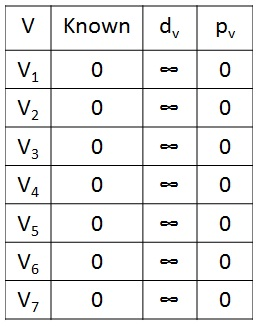Fig 1. Example Graph with initial configuration table

### Steps to find the shortest path

1. Initially cost associated with none of the vertices are known. So the entry in "known" value for a vertex is 0, dv is ∞ and pv is 0.
2. Select the source vertex 's' and set the known entry to '1'
3. Update the distance value of all adjacent vertices of the source vertex s by using

dw=dv + Cv, w

where Cv, w is the cost or weight on the edge of the adjacent vertex.
4. Next, pick up the vertex V who has the smallest distance dv among all the unknown vertices.
5. Then declare that the shortest path from s to v is known. Again update the distance value dv of all the adjacent vertices of v by using dw=dv + Cv, w.
6. If the new value dw is greater than the old one, then we simply ignore that value otherwise we will insert the new value into the dv entry.
7. Repeat the steps 3, 4 and 5 until all vertices are known.

### Finding Shortest path for Above Graph

Let us consider the above graph, now we want to find the shortest path from source to all other vertices using Dijkstra's algorithm.

#### Step 1

First, we select the source vertex as V1, with path length 0 and we set known value to 1 and update the distance value of adjacent vertices such as V2, V3, and V4.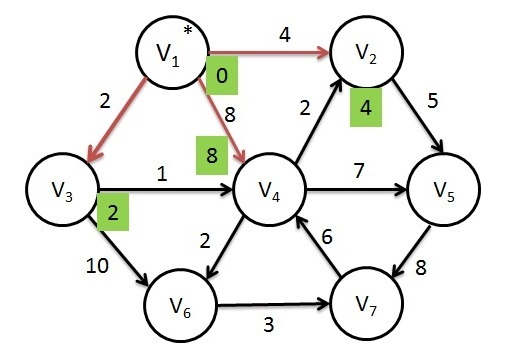Fig 2. After V1 is known

#### Step 2

Next, V3 is selected and set known value to 1 and update the adjacent vertices V4 and V6. Actually, V4 is already reached with cost of 8, but the cost of going through v3 will be 3 which is smaller than the previous cost. So we can replace that old one with new distance value.Fig 3. After V3 is known

#### Step 3

Next, V4 is selected and marked known. The vertices V2, V5 and V6 are adjacent. The vertex V2 is adjacent but not adjusted, because the cost of going via V4 is 3+2=5 which is greater than the previous distance 4. So simply discards the new value. Similarly for V6, the cost is changed to 5.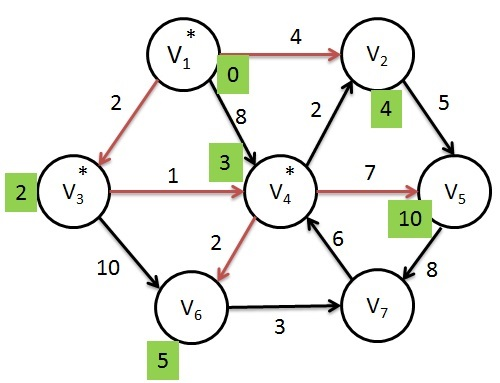Fig 4. After V4 is known

#### Step 4

Next, V2 is selected and marked known. V5 is the only adjacent vertex which is adjusted to 9(4+5) < 10.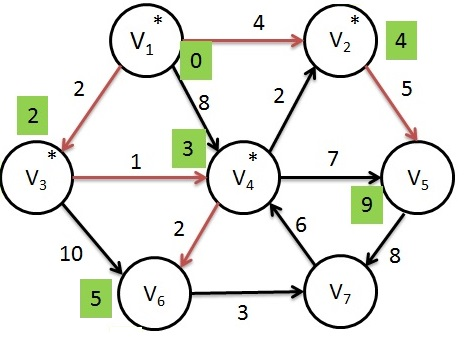Fig 5. After V2 is known

#### Step 5

Next, selected vertex is V6 and marked known. V7 is the only adjacent vertex which is adjusted to 8(5+3).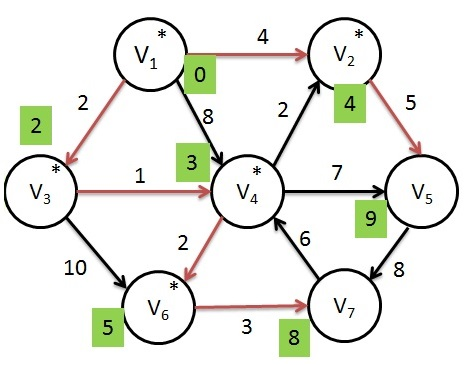Fig 6. After V6 is known

#### Step 6

Next, selected vertex is V7 and set known to 1. V4 is adjacent but already known, so no work is done on it.Fig 7. After V7 is known

#### Step 7

Finally, V5 is selected and marked known. Now the Dijkstra's algorithm is terminated and print out the shortest path from a start vertex to all other vertices.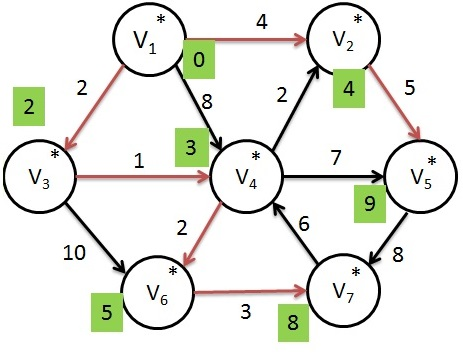Fig 8. After V5 is known

# Dijkstra's Algorithms

## Dijkstra's Algorithm - Shortest Path First Algorithm

Dijkstra's algorithm used to solve single source shortest path problem. It follows a Greedy Algorithm. Generally greedy algorithm solves a problem in stages and it finds the solution that appears to be the optimum solution at each stage. The Dijkstra's algorithm proceeds in stage. At each stage, it finds the shortest path from source to every other vertex. For doing this, we will keep track of three pieces of information for each vertex such as

• Known = set to 1 for a vertex after it is processed. Initially all vertices are not known.
• dv = keeps distance of the vertex from source s. Initially all vertices are ∞ except source s.
• pv = book keeping variable, used to print actual path.Fig 1. Example Graph with initial configuration table

### Steps to find the shortest path

1. Initially cost associated with none of the vertices are known. So the entry in "known" value for a vertex is 0, dv is ∞ and pv is 0.
2. Select the source vertex 's' and set the known entry to '1'
3. Update the distance value of all adjacent vertices of the source vertex s by using

dw=dv + Cv, w

where Cv, w is the cost or weight on the edge of the adjacent vertex.
4. Next, pick up the vertex V who has the smallest distance dv among all the unknown vertices.
5. Then declare that the shortest path from s to v is known. Again update the distance value dv of all the adjacent vertices of v by using dw=dv + Cv, w.
6. If the new value dw is greater than the old one, then we simply ignore that value otherwise we will insert the new value into the dv entry.
7. Repeat the steps 3, 4 and 5 until all vertices are known.

### Finding Shortest path for Above Graph

Let us consider the above graph, now we want to find the shortest path from source to all other vertices using Dijkstra's algorithm.

#### Step 1

First, we select the source vertex as V1, with path length 0 and we set known value to 1 and update the distance value of adjacent vertices such as V2, V3, and V4.Fig 2. After V1 is known

#### Step 2

Next, V3 is selected and set known value to 1 and update the adjacent vertices V4 and V6. Actually, V4 is already reached with cost of 8, but the cost of going through v3 will be 3 which is smaller than the previous cost. So we can replace that old one with new distance value.Fig 3. After V3 is known

#### Step 3

Next, V4 is selected and marked known. The vertices V2, V5 and V6 are adjacent. The vertex V2 is adjacent but not adjusted, because the cost of going via V4 is 3+2=5 which is greater than the previous distance 4. So simply discards the new value. Similarly for V6, the cost is changed to 5.Fig 4. After V4 is known

#### Step 4

Next, V2 is selected and marked known. V5 is the only adjacent vertex which is adjusted to 9(4+5) < 10.Fig 5. After V2 is known

#### Step 5

Next, selected vertex is V6 and marked known. V7 is the only adjacent vertex which is adjusted to 8(5+3).Fig 6. After V6 is known

#### Step 6

Next, selected vertex is V7 and set known to 1. V4 is adjacent but already known, so no work is done on it.Fig 7. After V7 is known

#### Step 7

Finally, V5 is selected and marked known. Now the Dijkstra's algorithm is terminated and print out the shortest path from a start vertex to all other vertices.Fig 8. After V5 is known

### << Previous Data Structures Home >>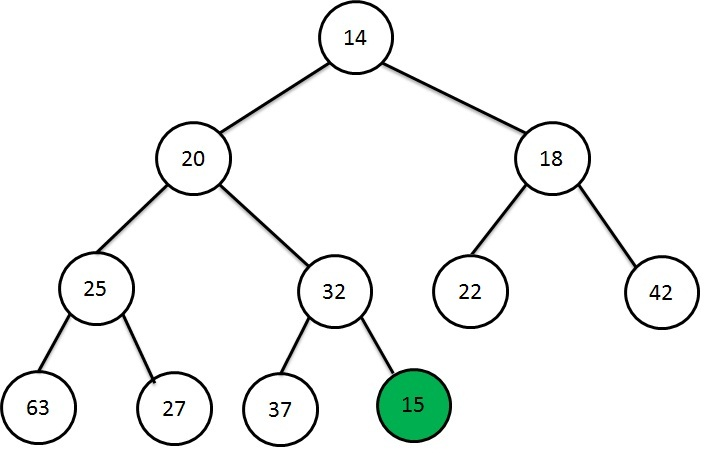Heap Data Structure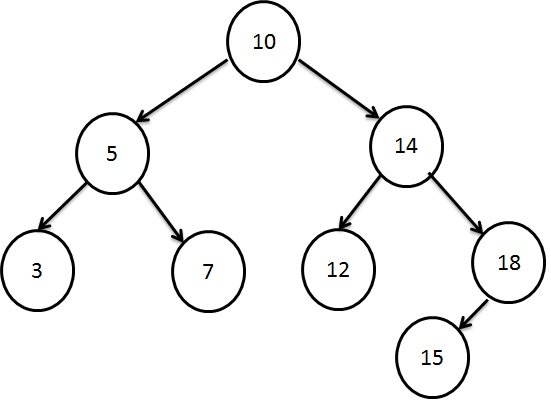Searching using Binary Search Tree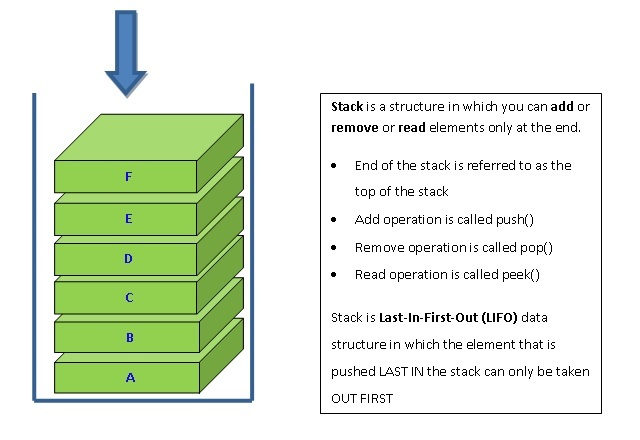Stacking the Data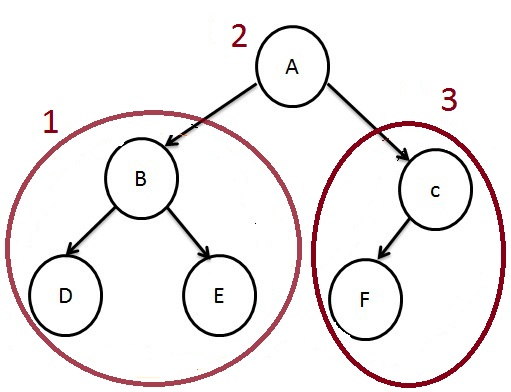How to traverse a Tree?